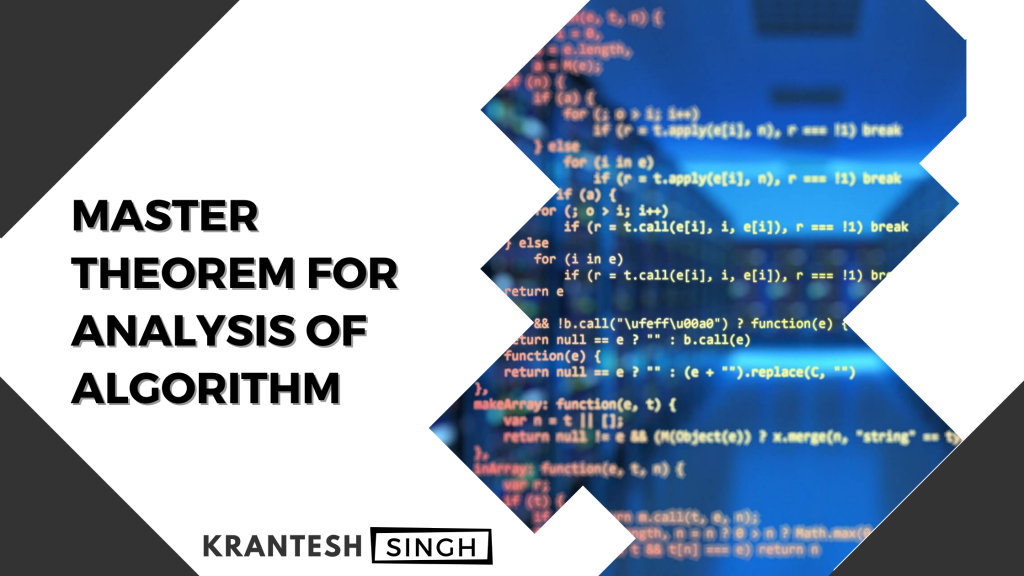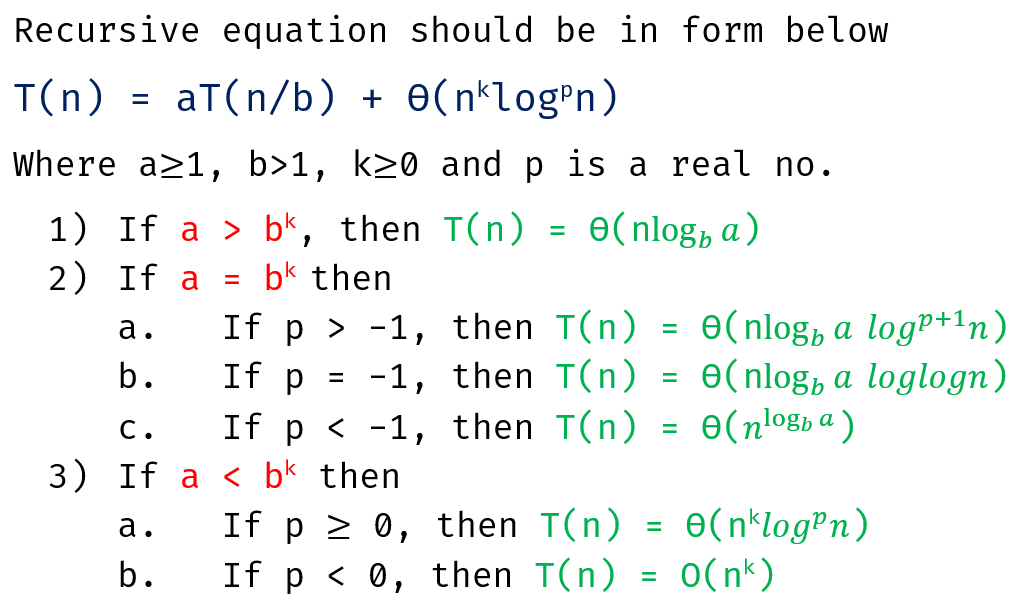Krantesh Singh# Master Theorem for Analysis of Algorithm

If you’re a computer science student or a programmer you may be familiar with term algorithm and time complexity related to a computer program so in this article you’re going to learn about MASTER THEOREM – Analysis of Algorithm.

We use master theorem for given recursive equation. If we have recursive equation then by using master’s theorem we can easily find out the time complexity of the recursive equation of a program. generally finding time complexity is tidious task but with master’s theorem you’ll be master in it.

Everything comes with certain conditions so using master theorem is not completely straight forward it has certain limitations and conditions.Above you can see the master’s theorem with its general form of equation and various conditions of a, b, k with with we have to check certain conditions to find out the time complexity.

Also we will take some problems related to finding time complexity of recursive equations so that you will find it easy to understand the master theorem and implement it.